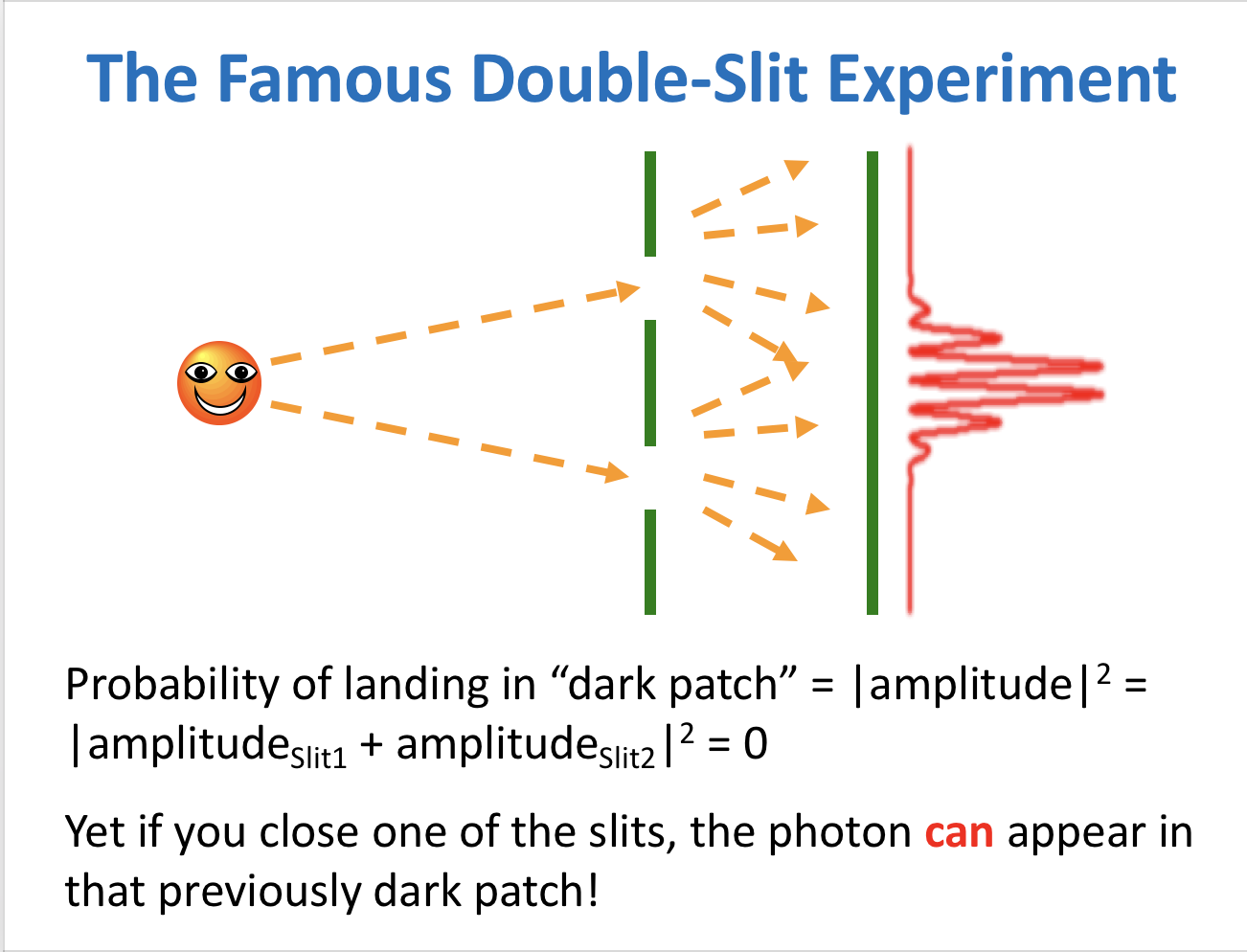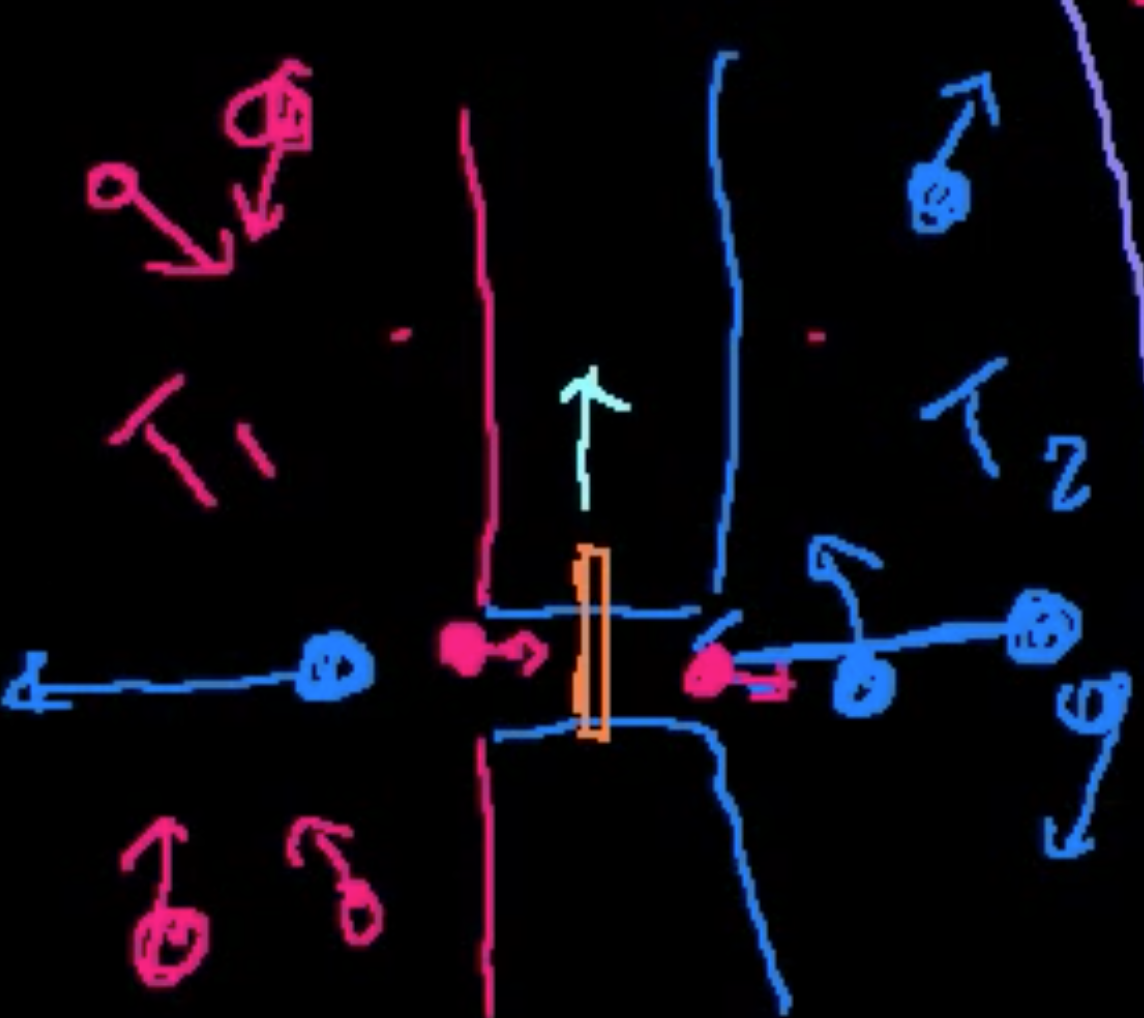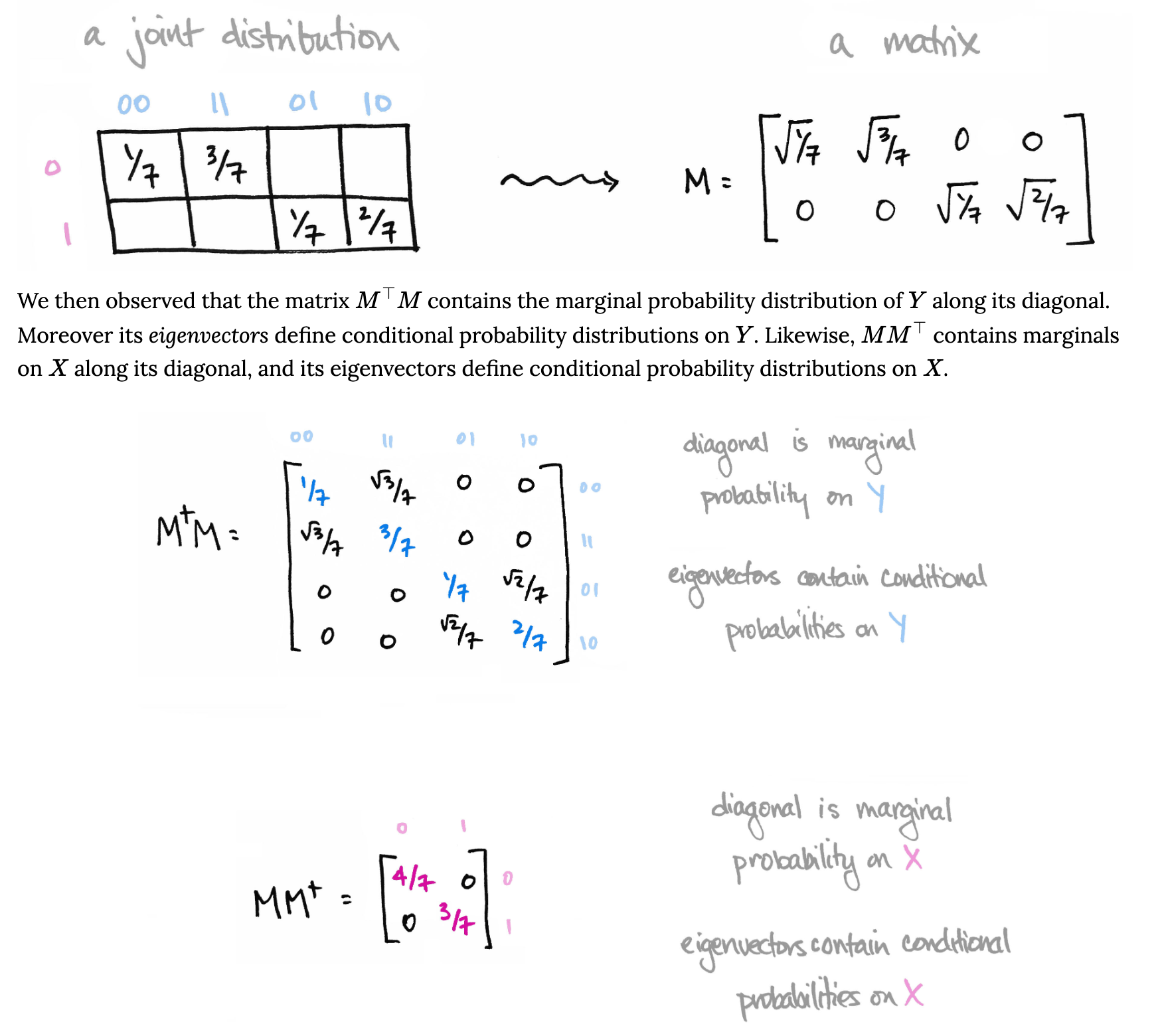quantum

Some very limited notes on quantum computing

• what does physics tell us about the limits of computers?
• NP - can check soln in polynomial time
• NP-hard - if solved, solves every NP
• NP-complete - NP hard and in NP
• church-turing thesis $\implies$ turing machine polynomial time should be best possible in the universe
• physics could allow us to do better than a turing machine
• examples
• glass plates with soapy water - forms minimum steiner tree
• can get stuck in local optimum
• ex. protein folding
• ex. relativity computer
• leave computer on earth, travel at speed of light for a while, come back and should be done
• if you want exponential speedup, need to get exponentially close to speed of light (requires exponential energy)
• ex. zeno’s computer - run clock faster (exponentially more cooling = energy)

# basics

• An n-bit computer has 2^n states and is in one of them with probability 1. You can think of it as having 2^n coefficients, one of which is 0 and the rest of which are 1. Operations on it are multiplying these coefficients by stochastic matrices. Only produces n bits of info.
• an n-qubit quantum computer is described by 2^n complex coefficients. The sum of their squares sums to 1. It’s 2^n complex coefficients must be multiplied by unitary matrices (they preserve that the sum of the squares add up to 1.)
• Problem: Decoherence – results from interaction with the outside world
• Properties:
• Superposition – an object is in more than one state at once
• Has a percentage of being in both states
• Entanglement – 2 particles behave exactly the opposite – instantly

# storing qubits

• Fullerenes – naturally found in Precambrian rock, reasonable for storing qubits – can store
• not developed, but some experiments have shown ability to store qubits for milliseconds

# intro

• probability with minus signs
• amplitudes - used to calculate probabilites, but can be negative / complex• applications
• quantum simulation
• also could factor integers in polynomial time (shor 1994)
• scaling up is hard because of decoherence= interaction between cubits and outside world
• error-correcting codes can make it so we can still work with some decoherence
• algorithms
• paths that lead to wrong answer - quantum amplitudes cancel each other out
• for right answer, quantum amplitudes in phase (all positive or all negative)
• prime factorization is NP but not NP complete
• unclear that quantum can solve all NP problems
• Grover’s algorithm - with quantum computers, something like you can only use sqrt of number of steps
• adiabatic optimization - like quantum simulated annealing, maybe can solve NP-complete problems
• dwave - company made ~2000 cubit machine
• don’t maintain coherence well
• algorithms for NP-complete problems may not work
• hope: quantum tunneling can get past local maximum in polynomial time maybe
• empircally unclear if this is true
• quantum supremacy - getting quantum speedup for something, maybe not something useful

# maxwell’s demon

• second law of thermodynamics: entropy is always increasing
• hot things transfer heat to cold things
• temperature is avg kinetic energy - particles follow a diistribution of temperature
• separate 2 samples (one hot, one cold) with insulator
• idea: demon makes all fast particles go to hot side, all slow particles go to slow side - this is against entropy
• demon controls door between the samples
•• demon opens door whenever high temperature particle comes from cold sample, then closes
• demon opens door for slow particles from hot sample, then closes
• problem: demon has to track all the particles (which would generate a lot of heat)

# quantum probability

• based on this blog post

• marginal prob. loses information but we don’t need to
•